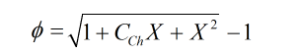# C311 – ISO 5167/TR 12748 Wet Gas Orifice Flow Calculation

## Description

This calculates the corrected dry gas mass flow rate for orifice plate metering systems handling a wet gas flow. The formulae from ISO TR12748 are applied to the ISO 5167 calculated dry gas mass flow rate in order to correct the over reading that occurs when a wet gas is encountered.

ISO TR 12748:2015 – Natural Gas – Wet gas flow measurement in natural gas operations

Horizontally Installed Orifice Plate Meter Response to Wet Gas Flows – A.Hall, B.Priddy, R.Steven, G.Stobie (2011)

ISO 5167: Measurement of fluid flow by means of pressure differential devices inserted in circular cross-section conduits running full

ISO TR9464: Guidelines for the use of ISO 5167

Kelton calculation reference C311

FLOCALC calculation reference F072

Kelton calculation C270 – ISO 5167 Orifice Mass Flow Calculation

FLOCALC calculation F037: Upstream Density Calculation

## Options

### Tapping Type

• Flange
• D/D2
• Corner

This option group allows the selection of the tapping type used for the pressure measurements on the orifice plate system.

### Expansibility factor

• User entered
• Calculated

This option give the choice of calculating expansibility or entering a keypad value.

### Temperature

• Upstream
• Downstream

This option allows selection of where the temperature is measured, upstream of the orifice plate meter or downstream where the flow has fully recovered.

### Joule-Thomson Coefficient

• User entered
• Calculated

This option allows the Joule-Thomson coefficient to be entered if the value is known or calculated by Reader-Harris’ simplified equation (ISO TR9494:2008) if otherwise.

### Density

• Upstream
• Downstream

This option group allows selection where the density measurement is taken, upstream of the orifice plate or downstream at pressure, p2, and temperature T3.

### Density Referral

• Isentropic
• Isenthalpic

This option group will appear if the downstream density option is selected. It allows a choice of whether density is corrected to upstream conditions using an isentropic or isenthalpic (PTZ) correction as shown in F037.

### Liquid

• Hydrocarbon
• Water
• Water-hydrocarbon mixture

This option is used to specify the nature of the liquid within the flow.

### Liquid density

• User entered
• Calculated

This option is used to select whether to input the liquid density or calculate it from known water and hydrocarbon liquid densities as well as water to liquid mass ratio.

### Chisholm exponent (n)

• User entered
• Calculated

This option is used to specify whether the n value used is calculated as per the standard or user entered. Note n has a (editable) default of 0.25 if user entered is selected as this is the value from Chisholm’s original correlation.

## Calculation

### Uncorrected Mass Flow Rate

The uncorrected mass flow rate is calculated by: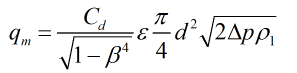Where Cd = Coefficient of discharge β = Diameter ratio ε = Expansibility factor d = Orifice bore Δp = Differential pressure ρ1 = Upstream density

### Coefficient of discharge

The Reader-Harris/Gallagher equation is used to calculate dry gas discharge coefficient.

### Expansibility Factor

The expansibility factor is calculated by: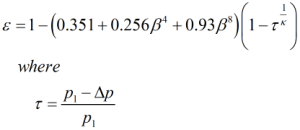### Pressure Loss

The permanent pressure loss is calculated by: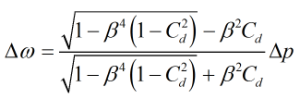### Temperature Referral

Upstream temperature is calculated by: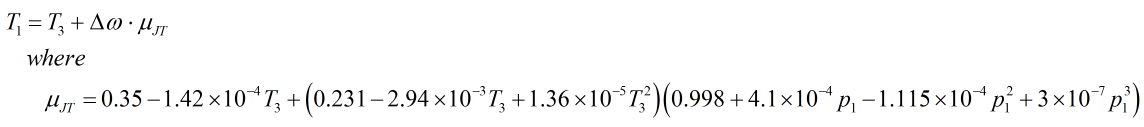Where p1 = Upstream pressure κ = Isentropic exponent T3 = Recovery point temperature Δω = Permanent pressure loss μJT = Joule-Thomson coefficient

0

### Liquid density

If liquid density is calculated it done by: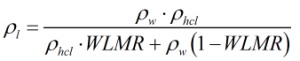Where WLMR = Water liquid mass ratio ρw = Density of water ρhcl = Density of hydrocarbon liquid

### Lockhart-Martinelli parameter

The Lockhart-Martinelli parameter is calculated by: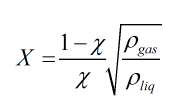Where χ = Gas mass fraction ρliq = Density of the liquid ρgas = Density of the gas

### Chisholm coefficient

The Chisholm coefficient is calculated by: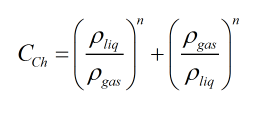Where n = Chisholm exponent ρliq = Density of the liquid ρgas = Density of the gas

### Chisholm exponent

If the Chisholm exponent is calculated it is done by: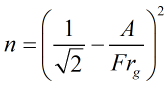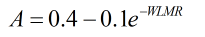Where Frg = Gas Froude number